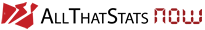# Definition

"The GDP deflator (implicit price deflator for GDP) is calculated as GDP at current prices divided by GDP at “constant prices” (chained volume estimates or fixed-base volume estimates, depending on countries)." OECD, Paris

# Example

You will find the GDP deflator e.g. here: DSI's Global Economic Statistics.

But it's also possible to calculate it manually: download the GDP at current prices and GDP at constant prices for the country of your choice, here: Saudi Arabia, currency: Saudi Riyal

Do the math requested by definition:

GDP deflator for Saudi Riyal = GDP at current prices / GDP at constant prices

All graphics and tables are created with